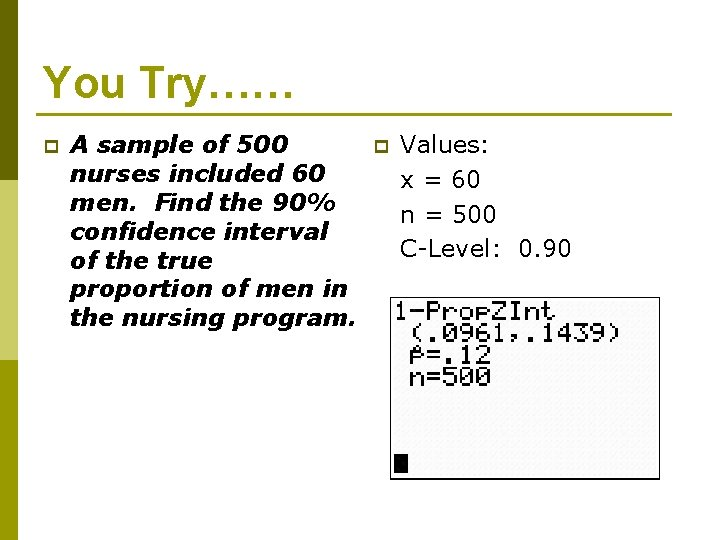# Confidence Intervals Using the TI84 Objective Find confidence

• Slides: 15Confidence Intervals Using the TI-84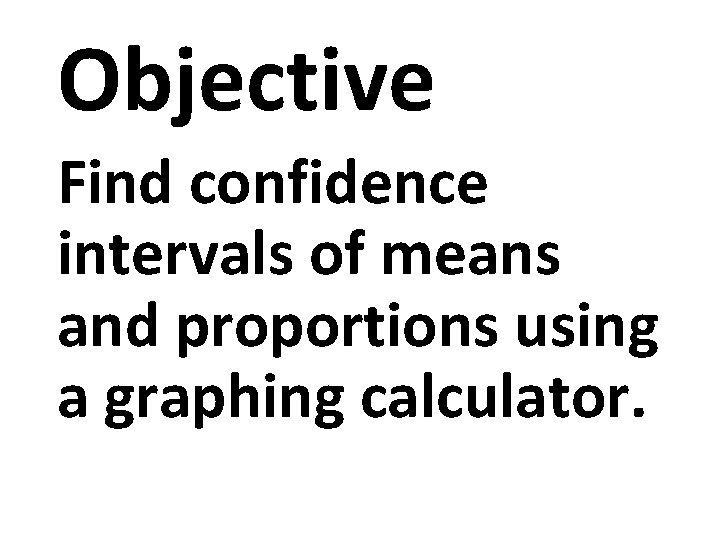Objective Find confidence intervals of means and proportions using a graphing calculator.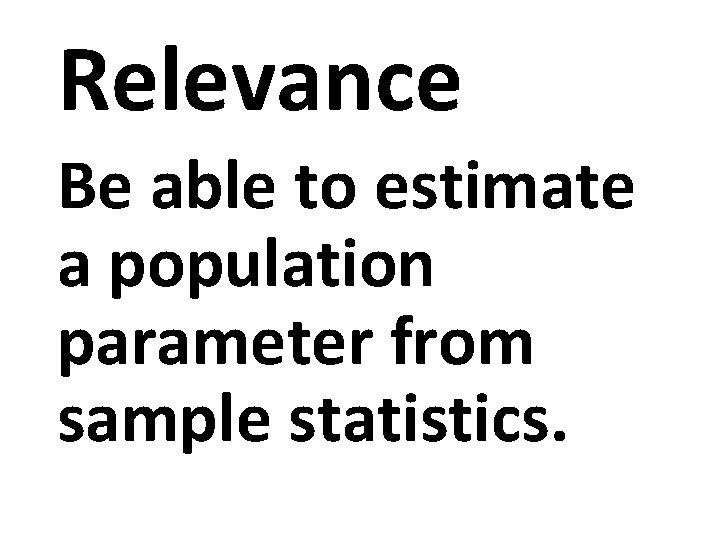Relevance Be able to estimate a population parameter from sample statistics.p Let’s go back to confidence intervals for one sample and learn how to find an interval using our TI-84’s. p On the final exam, you will have to do confidence intervals. For the sake of time, you are encouraged to use the calculator.Z-Intervals…… p A study of 40 math teachers showed that they spent, on average, 12. 6 minutes correcting papers. Find the 90% confidence interval of the mean time of correcting papers if the st. deviation is 2. 5 minutes. p Values:p 1. 2. 3. 4. 5. Calculator Steps: Stat Tests 7: ZInterval Set on Stats if you are going to input the data yourself. Set on data if your data is in a list. Fill in and calculate.You Try…… p A random sample of 49 female shoppers showed that they spent an average of \$23. 45 per visit at a grocery store. The st. deviation was \$2. 80. Find the 95% confidence interval of the mean.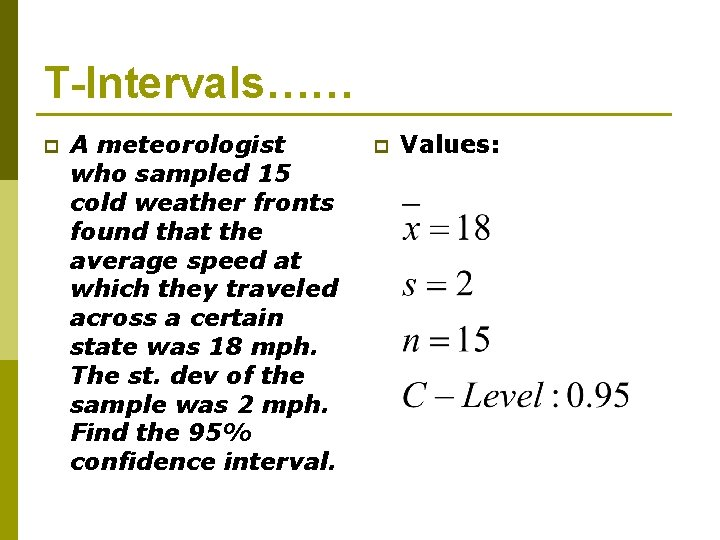T-Intervals…… p A meteorologist who sampled 15 cold weather fronts found that the average speed at which they traveled across a certain state was 18 mph. The st. dev of the sample was 2 mph. Find the 95% confidence interval. p Values: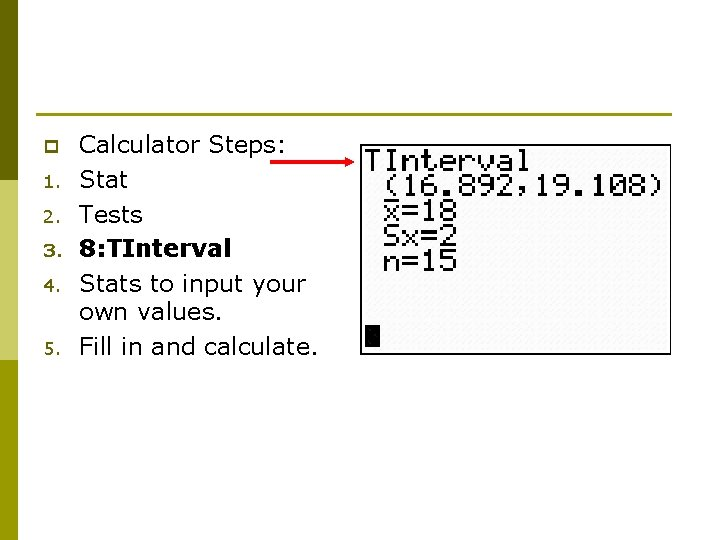p 1. 2. 3. 4. 5. Calculator Steps: Stat Tests 8: TInterval Stats to input your own values. Fill in and calculate.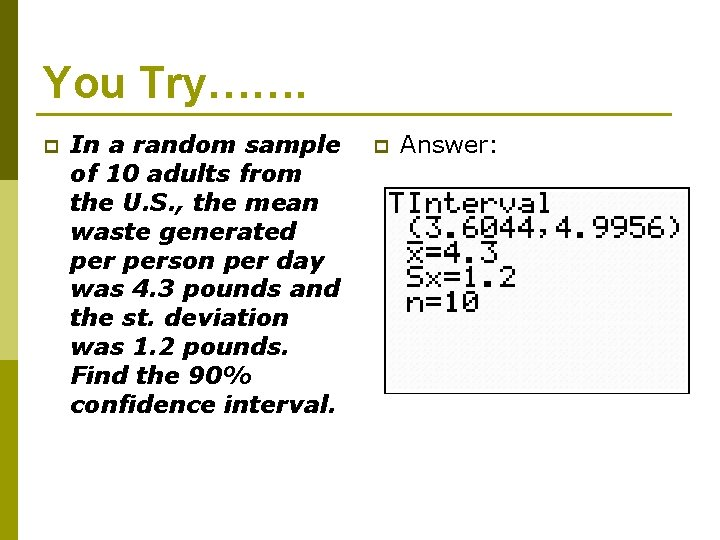You Try……. p In a random sample of 10 adults from the U. S. , the mean waste generated person per day was 4. 3 pounds and the st. deviation was 1. 2 pounds. Find the 90% confidence interval. p Answer: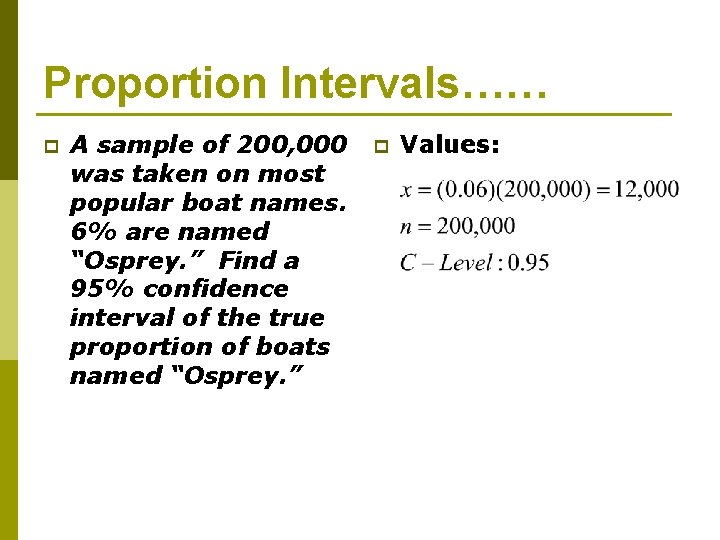Proportion Intervals…… p A sample of 200, 000 was taken on most popular boat names. 6% are named “Osprey. ” Find a 95% confidence interval of the true proportion of boats named “Osprey. ” p Values: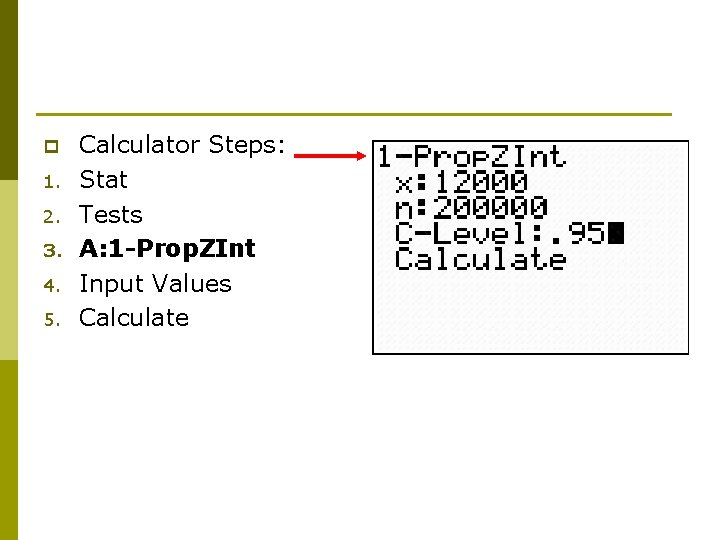p 1. 2. 3. 4. 5. Calculator Steps: Stat Tests A: 1 -Prop. ZInt Input Values Calculate